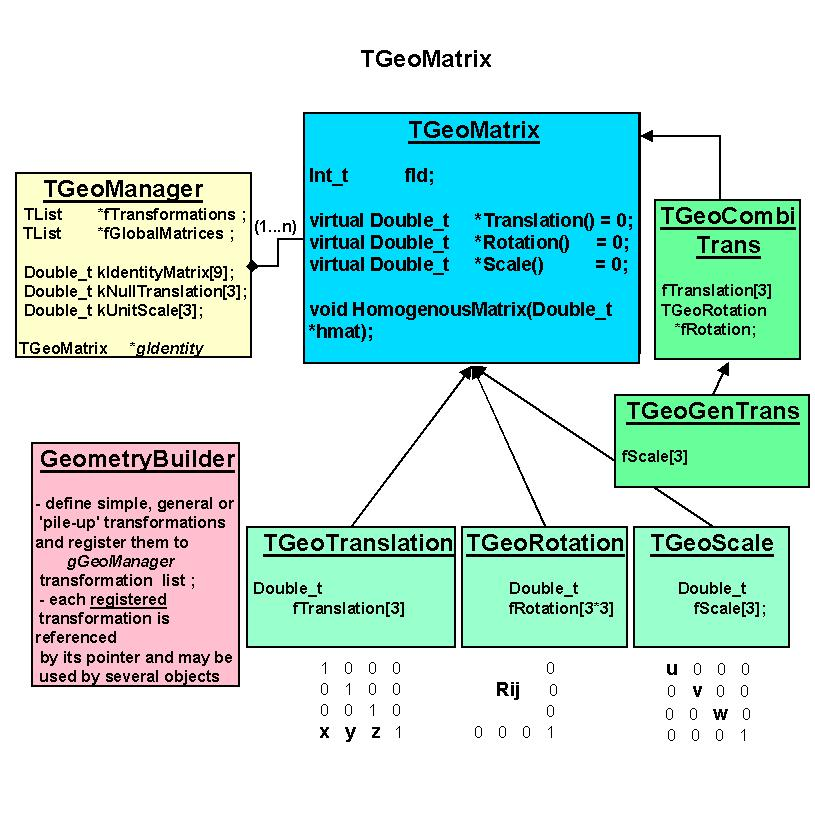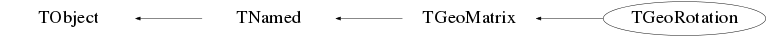# class TGeoRotation: public TGeoMatrix

``` Geometrical transformation package.

All geometrical transformations handled by the modeller are provided as a
built-in package. This was designed to minimize memory requirements and
optimize performance of point/vector master-to-local and local-to-master
computation. We need to have in mind that a transformation in TGeo has 2
major use-cases. The first one is for defining the placement of a volume
with respect to its container reference frame. This frame will be called
'master' and the frame of the positioned volume - 'local'. If T is a
transformation used for positioning volume daughters, then:

MASTER = T * LOCAL

Therefore a local-to-master conversion will be performed by using T, while
a master-to-local by using its inverse. The second use case is the computation
of the global transformation of a given object in the geometry. Since the
geometry is built as 'volumes-inside-volumes', this global transformation
represent the pile-up of all local transformations in the corresponding
branch. The conversion from the global reference frame and the given object
is also called master-to-local, but it is handled by the manager class.
A general homogenous transformation is defined as a 4x4 matrix embeeding
a rotation, a translation and a scale. The advantage of this description
is that each basic transformation can be represented as a homogenous matrix,
composition being performed as simple matrix multiplication.
Rotation:                      Inverse rotation:
r11  r12  r13   0              r11  r21  r31   0
r21  r22  r23   0              r12  r22  r32   0
r31  r32  r33   0              r13  r23  r33   0
0    0    0    1               0    0    0    1

Translation:                   Inverse translation:
1    0    0    tx               1    0    0   -tx
0    1    0    ty               0    1    0   -ty
0    0    1    tz               0    0    1   -tz
0    0    0    1                0    0    0   1

Scale:                         Inverse scale:
sx   0    0    0              1/sx  0    0    0
0    sy   0    0               0   1/sy  0    0
0    0    sz   0               0    0   1/sz  0
0    0    0    1               0    0    0    1

where: rij are the 3x3 rotation matrix components,
tx, ty, tz are the translation components
sx, sy, sz are arbitrary scale constants on the eacks axis,

The disadvantage in using this approach is that computation for 4x4 matrices
is expensive. Even combining two translation would become a multiplication
of their corresponding matrices, which is quite an undesired effect. On the
other hand, it is not a good idea to store a translation as a block of 16
numbers. We have therefore chosen to implement each basic transformation type
as a class deriving from the same basic abstract class and handling its specific
data and point/vector transformation algorithms.

```
/**/
```
The base class TGeoMatrix defines abstract metods for:

- translation, rotation and scale getters. Every derived class stores only
its specific data, e.g. a translation stores an array of 3 doubles and a
rotation an array of 9. However, asking which is the rotation array of a
TGeoTranslation through the base TGeoMatrix interface is a legal operation.
The answer in this case is a pointer to a global constant array representing
an identity rotation.
Double_t *TGeoMatrix::GetTranslation()
Double_t *TGeoMatrix::GetRotation()
Double_t *TGeoMatrix::GetScale()

- MasterToLocal() and LocalToMaster() point and vector transformations :
void      TGeoMatrix::MasterToLocal(const Double_t *master, Double_t *local)
void      TGeoMatrix::LocalToMaster(const Double_t *local, Double_t *master)
void      TGeoMatrix::MasterToLocalVect(const Double_t *master, Double_t *local)
void      TGeoMatrix::LocalToMasterVect(const Double_t *local, Double_t *master)
These allow correct conversion also for reflections.
- Transformation type getters :
Bool_t    TGeoMatrix::IsIdentity()
Bool_t    TGeoMatrix::IsTranslation()
Bool_t    TGeoMatrix::IsRotation()
Bool_t    TGeoMatrix::IsScale()
Bool_t    TGeoMatrix::IsCombi() (translation + rotation)
Bool_t    TGeoMatrix::IsGeneral() (translation + rotation + scale)

Combinations of basic transformations are represented by specific classes
deriving from TGeoMatrix. In order to define a matrix as a combination of several
others, a special class TGeoHMatrix is provided. Here is an example of matrix
creation :

Matrix creation example:

root TGeoRotation r1,r2;
r1.SetAngles(90,0,30);        // rotation defined by Euler angles
r2.SetAngles(90,90,90,180,0,0); // rotation defined by GEANT3 angles
TGeoTranslation t1(-10,10,0);
TGeoTranslation t2(10,-10,5);
TGeoCombiTrans c1(t1,r1);
TGeoCombiTrans c2(t2,r2);
TGeoHMatrix h = c1 * c2; // composition is done via TGeoHMatrix class
root TGeoHMatrix *ph = new TGeoHMatrix(hm); // this is the one we want to
// use for positioning a volume
root ph->Print();

pVolume->AddNode(pVolDaughter,id,ph) // now ph is owned by the manager

Rule for matrix creation:
- unless explicitly used for positioning nodes (TGeoVolume::AddNode()) all
matrices deletion have to be managed by users. Matrices passed to geometry
have to be created by using new() operator and their deletion is done by
TGeoManager class.

Available geometrical transformations

1. TGeoTranslation - represent a (dx,dy,dz) translation. Data members:
Double_t fTranslation. Translations can be added/subtracted.
TGeoTranslation t1;
t1->SetTranslation(-5,10,4);
TGeoTranslation *t2 = new TGeoTranslation(4,3,10);
t2->Subtract(&t1);

2. Rotations - represent a pure rotation. Data members: Double_t fRotationMatrix[3*3].
Rotations can be defined either by Euler angles, either, by GEANT3 angles :
TGeoRotation *r1 = new TGeoRotation();
r1->SetAngles(phi, theta, psi); // all angles in degrees
This represent the composition of : first a rotation about Z axis with
angle phi, then a rotation with theta about the rotated X axis, and
finally a rotation with psi about the new Z axis.

r1->SetAngles(th1,phi1, th2,phi2, th3,phi3)
This is a rotation defined in GEANT3 style. Theta and phi are the spherical
angles of each axis of the rotated coordinate system with respect to the
initial one. This construction allows definition of malformed rotations,
e.g. not orthogonal. A check is performed and an error message is issued
in this case.

Specific utilities : determinant, inverse.

3. Scale transformations - represent a scale shrinking/enlargement. Data
members :Double_t fScale. Not fully implemented yet.

4. Combined transformations - represent a rotation folowed by a translation.
Data members: Double_t fTranslation, TGeoRotation *fRotation.
TGeoRotation *rot = new TGeoRotation("rot",10,20,30);
TGeoTranslation trans;

TGeoCombiTrans *c1 = new TGeoCombiTrans(trans, rot);
TGeoCombiTrans *c2 = new TGeoCombiTrans("somename",10,20,30,rot)

5. TGeoGenTrans - combined transformations including a scale. Not implemented.
6. TGeoIdentity - a generic singleton matrix representing a identity transformation
NOTE: identified by the global variable gGeoIdentity.

```

## Function Members (Methods)

public:
protected:
 void CheckMatrix() virtual void TObject::DoError(int level, const char* location, const char* fmt, va_list va) const void TObject::MakeZombie()

## Data Members

public:
 enum TGeoMatrix::EGeoTransfTypes { kGeoIdentity kGeoShared kGeoTranslation kGeoRotation kGeoScale kGeoReflection kGeoRegistered kGeoSavePrimitive kGeoMatrixOwned kGeoCombiTrans kGeoGenTrans }; enum TObject::EStatusBits { kCanDelete kMustCleanup kObjInCanvas kIsReferenced kHasUUID kCannotPick kNoContextMenu kInvalidObject }; enum TObject::[unnamed] { kIsOnHeap kNotDeleted kZombie kBitMask kSingleKey kOverwrite kWriteDelete };
protected:
 TString TNamed::fName object identifier Double_t fRotationMatrix rotation matrix TString TNamed::fTitle object title

## Class Charts## Function documentation

``` Default constructor.
```
TGeoRotation(const TGeoRotation& other)
``` Copy ctor.
```
TGeoRotation(const TGeoMatrix& other)
``` Copy ctor.
```
TGeoRotation(const char* name)
``` Named rotation constructor
```
TGeoRotation(const char* name, Double_t phi, Double_t theta, Double_t psi)
``` Default rotation constructor with Euler angles. Phi is the rotation angle about
Z axis  and is done first, theta is the rotation about new Y and is done
second, psi is the rotation angle about new Z and is done third. All angles are in
degrees.
```
TGeoRotation(const char* name, Double_t theta1, Double_t phi1, Double_t theta2, Double_t phi2, Double_t theta3, Double_t phi3)
``` Rotation constructor a la GEANT3. Angles theta(i), phi(i) are the polar and azimuthal
angles of the (i) axis of the rotated system with respect to the initial non-rotated
system.
Example : the identity matrix (no rotation) is composed by
theta1=90, phi1=0, theta2=90, phi2=90, theta3=0, phi3=0
SetBit(kGeoRotation);
```
TGeoMatrix& Inverse() const
``` Return a temporary inverse of this.
```
Bool_t IsValid() const
``` Perform orthogonality test for rotation.
```
void Clear(Option_t* option = "")
``` reset data members
```
void FastRotZ(const Double_t* sincos)
``` Perform a rotation about Z having the sine/cosine of the rotation angle.
```
Double_t GetPhiRotation(Bool_t fixX = kFALSE) const
```--- Returns rotation angle about Z axis in degrees. If the rotation is a pure
rotation about Z, fixX parameter does not matter, otherwise its meaning is:
- fixX = true  : result is the phi angle of the projection of the rotated X axis in the un-rotated XY
- fixX = false : result is the phi angle of the projection of the rotated Y axis - 90 degrees
```
void LocalToMaster(const Double_t* local, Double_t* master) const
``` convert a point by multiplying its column vector (x, y, z, 1) to matrix inverse
```
void MasterToLocal(const Double_t* master, Double_t* local) const
``` convert a point by multiplying its column vector (x, y, z, 1) to matrix
```
TGeoMatrix * MakeClone() const
``` Make a clone of this matrix.
```
void RotateX(Double_t angle)
``` Rotate about X axis of the master frame with angle expressed in degrees.
```
void RotateY(Double_t angle)
``` Rotate about Y axis of the master frame with angle expressed in degrees.
```
void RotateZ(Double_t angle)
``` Rotate about Z axis of the master frame with angle expressed in degrees.
```
void ReflectX(Bool_t leftside, Bool_t rotonly = kFALSE)
``` Multiply by a reflection respect to YZ.
```
void ReflectY(Bool_t leftside, Bool_t rotonly = kFALSE)
``` Multiply by a reflection respect to ZX.
```
void ReflectZ(Bool_t leftside, Bool_t rotonly = kFALSE)
``` Multiply by a reflection respect to XY.
```
void SavePrimitive(ostream& out, Option_t* option = "")
``` Save a primitive as a C++ statement(s) on output stream "out".
```
void SetRotation(const TGeoMatrix& other)
``` Copy rotation elements from other rotation matrix.
```
void SetAngles(Double_t phi, Double_t theta, Double_t psi)
``` Set matrix elements according to Euler angles
```
void SetAngles(Double_t theta1, Double_t phi1, Double_t theta2, Double_t phi2, Double_t theta3, Double_t phi3)
``` Set matrix elements in the GEANT3 way
```
void GetAngles(Double_t& theta1, Double_t& phi1, Double_t& theta2, Double_t& phi2, Double_t& theta3, Double_t& phi3) const
``` Retreive rotation angles
```
void GetAngles(Double_t& phi, Double_t& theta, Double_t& psi) const
``` Retreive Euler angles.
```
Double_t Determinant() const
``` computes determinant of the rotation matrix
```
void CheckMatrix()
``` performes an orthogonality check and finds if the matrix is a reflection
Warning("CheckMatrix", "orthogonality check not performed yet");
```
void GetInverse(Double_t* invmat) const
``` Get the inverse rotation matrix (which is simply the transpose)
```
void MultiplyBy(TGeoRotation* rot, Bool_t after = kTRUE)
``` Multiply this rotation with the one specified by ROT.
-   after=TRUE (default): THIS*ROT
-   after=FALSE         : ROT*THIS
```
TGeoMatrix& operator=(const TGeoMatrix& matrix)
``` Preventing warnings with -Weffc++ in GCC since the behaviour of operator * was chosen so by design.
```
const Double_t * GetTranslation() const
const Double_t * GetRotationMatrix() const
const Double_t * GetScale() const
void LocalToMasterVect(const Double_t* local, Double_t* master) const
void LocalToMasterBomb(const Double_t* local, Double_t* master) const
void MasterToLocalVect(const Double_t* master, Double_t* local) const
void MasterToLocalBomb(const Double_t* master, Double_t* local) const
TGeoTranslation& operator=(const TGeoMatrix& matrix)
virtual ~TGeoRotation()
`{}`
void SetMatrix(const Double_t* rot)
`{memcpy(&fRotationMatrix, rot, 9*sizeof(Double_t));CheckMatrix();}`